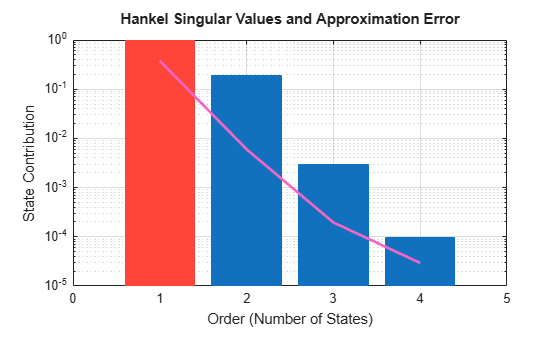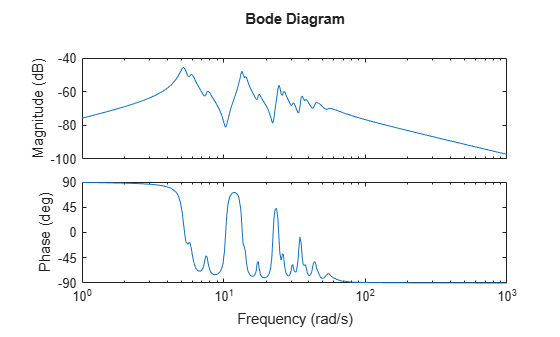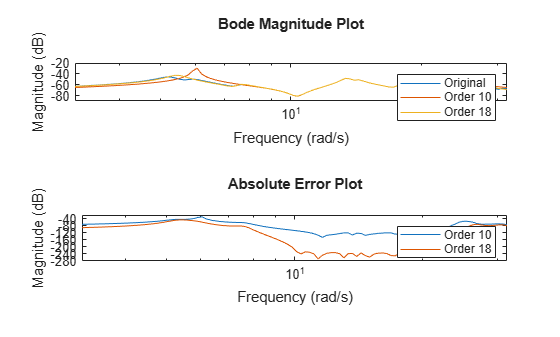Main Content

# balredOptions

Create option set for model order reduction

## Syntax

```opts = balredOptions opts = balredOptions('OptionName', OptionValue) ```

## Description

`opts = balredOptions` returns the default option set for the `balred` command.

`opts = balredOptions('OptionName', OptionValue)` accepts one or more comma-separated name/value pairs. Specify `OptionName` inside single quotes.

## Input Arguments

### Name-Value Pair Arguments

`'StateProjection'`

State elimination method. Specifies how to eliminate the weakly coupled states (states with smallest Hankel singular values). Specified as one of the following values:

 `'MatchDC'` Discards the specified states and alters the remaining states to preserve the DC gain. `'Truncate'` Discards the specified states without altering the remaining states. This method tends to product a better approximation in the frequency domain, but the DC gains are not guaranteed to match.

Default: `'MatchDC'`

`'ErrorBound'`

Error bound type, specified as either '`absolute`' or '`relative`'. Set '`Errorbound`' to

• '`absolute`' to control the absolute error ${‖G-{G}_{r}‖}_{\infty }$.

• '`relative`' to control the relative error ${‖{G}^{-1}\left(G-{G}_{r}\right)‖}_{\infty }$.

Relative error gives a better match across frequency while absolute error emphasizes areas with most gain. For more information, see `getPeakGain`.

Default: '`absolute`'

`'Regularization'`

Regularization level value that ensures a well-defined relative error at all frequencies. When you set `ErrorBound` to '`relative`', `balred` reduces the model `[sys,⍴*I]` instead of `sys`. Set this option to '`auto`' to let `balred` pick a suitable regularization level `⍴` value. Specify a value $\text{\hspace{0.17em}}\rho \ge 0$ to override this default.

Default: '`auto`'

`'FreqIntervals'`

Frequency intervals for computing frequency-limited Hankel singular values, specified as a matrix with two columns. Each row specifies a frequency interval `[fmin fmax]`, where `fmin` and `fmax` are nonnegative frequencies, expressed in the frequency unit of the model. When identifying low-energy states to truncate, the software computes state contributions to system behavior in these frequency ranges only. For example:

• To restrict the computation to the range between 3 rad/s and 15 rad/s, assuming the frequency unit of the model is rad/s, set `FreqIntervals` to `[3 15]`.

• To restrict the computation to two frequency intervals, 3-15 rad/s and 40-60 rad/s, use `[3 15; 40 60]`.

• To specify all frequencies below a cutoff frequency `fcut`, use `[0 fcut]`.

• To specify all frequencies above the cutoff, use ```[fcut Inf]``` in continuous time, or `[fcut pi/Ts]` in discrete time, where `Ts` is the sample time of the model.

The default value, `[]`, imposes no frequency limitation and is equivalent to `[0 Inf]` in continuous time or `[0 pi/Ts]` in discrete time. However, if you specify a `TimeIntervals` value other than `[]`, then this limit overrides `FreqIntervals = []`. If you specify both a `TimeIntervals` value and a `FreqIntervals` value, then the computation uses the union of these intervals.

If `StateProjection = 'MatchDC'` (the default value), then `balred` attempts to match the DC gain of the original and reduced models, even if the specified frequency intervals exclude 0. This behavior might reduce the quality of the match in the specified intervals. To improve the match within frequency intervals that exclude 0, set ```StateProjection = 'Truncate'```.

If both the frequency and time intervals do include DC, you can still set `StateElimMethod = 'Truncate'` to improve the match at other frequencies and times.

Default: `[]`

`'TimeIntervals'`

Time intervals for computing time-limited Hankel singular values, specified as a matrix with two columns. Each row specifies a time interval `[tmin tmax]`, where `tmin` and `tmax` are nonnegative times, expressed in the time unit of the model. When identifying low-energy states to truncate, the software computes state contributions to the system’s impulse response in these time intervals only. For example:

• To restrict the computation to the range between 3 s and 15 s, assuming the time unit of the model is seconds, set `TimeIntervals` to ```[3 15]```.

• To restrict the computation to two time intervals, 3-15 s and 40-60 s, use `[3 15; 40 60]`.

• To specify all times from zero up to a cutoff time `tcut`, use `[0 tcut]`. To specify all times after the cutoff, use `[tcut Inf]`.

The default value, `[]`, imposes no time limitation and is equivalent to `[0 Inf]`. However, if you specify a `FreqIntervals` value other than `[]`, then this limit overrides `Timeintervals = []`. If you specify both a `TimeIntervals` value and a `FreqIntervals` value, then the computation uses the union of these intervals.

If `StateProjection = 'MatchDC'` (the default value), then `balred` attempts to match the DC gain of the original and reduced models, even if the specified time intervals exclude `Inf`. This behavior might reduce the quality of the match in the specified intervals. To improve the match within time intervals that exclude `Inf`, set `StateProjection = 'Truncate'`.

If both the frequency and time intervals do include DC, you can still set `StateProjection = 'Truncate'` to improve the match at other frequencies and times.

Default: `[]`

`'SepTol'`

Maximum loss of accuracy value in stable and unstable decomposition. For models with unstable poles, `balred` first extracts the stable dynamics using `stabsep`. Use '`SepTol`' to control the decomposition accuracy. Increasing '`SepTol`' helps separate nearby stable and unstable modes at the expense of accuracy. For more information, see `stabsepOptions`.

Default: `10`

`'Offset'`

Offset for the stable/unstable boundary. Positive scalar value. In the stable/unstable decomposition, the stable term includes only poles satisfying

• `Re(s) < -Offset * max(1,|Im(s)|)` (Continuous time)

• `|z| < 1 - Offset` (Discrete time)

Increase the value of `Offset` to treat poles close to the stability boundary as unstable.

Default: `1e-8`

For additional information on the options and how to use them, see the `balred` reference page.

## Examples

collapse all

Compute a reduced-order approximation of the system given by:

`$G\left(s\right)=\frac{\left(s+0.5\right)\left(s+1.1\right)\left(s+2.9\right)}{\left(s+{10}^{-6}\right)\left(s+1\right)\left(s+2\right)\left(s+3\right)}.$`

Create the model.

`sys = zpk([-0.5 -1.1 -2.9],[-1e-6 -2 -1 -3],1);`

Exclude the pole at $s=1{0}^{-6}$ from the stable term of the stable/unstable decomposition. To do so, set the `Offset` option of `balredOptions` to a value larger than the pole you want to exclude.

`opts = balredOptions('Offset',0.001,'StateProjection','Truncate');`

Visualize the Hankel singular values (HSV) and the approximation error.

`balred(sys,opts)`Observe that the first HSV is red which indicates that it is associated with an unstable mode.

Now, compute a second-order approximation with the specified options.

```[rsys,info] = balred(sys,2,opts); rsys```
```rsys = 0.99113 (s+0.5235) ------------------- (s+1e-06) (s+1.952) Continuous-time zero/pole/gain model. ```

Notice that the pole at `-1e-6` appears unchanged in the reduced model `rsys`.

Compare the responses of the original and reduced-order models.

`bodeplot(sys,rsys,'r--')`Observe that the bode response of the original model and the reduced-order model nearly match.

Reduce a high-order model with a focus on the dynamics in a particular frequency range.

Load a model and examine its frequency response.

```load('highOrderModel.mat','G') bodeplot(G)````G` is a 48th-order model with several large peak regions around 5.2 rad/s, 13.5 rad/s, and 24.5 rad/s, and smaller peaks scattered across many frequencies. Suppose that for your application you are only interested in the dynamics near the second large peak, between 10 rad/s and 22 rad/s. Focus the model reduction on the region of interest to obtain a good match with a low-order approximation. Use `balredOptions` to specify the frequency interval for `balred`.

```bopt = balredOptions('StateProjection','Truncate','FreqIntervals',[10,22]); GLim10 = balred(G,10,bopt); GLim18 = balred(G,18,bopt);```

Examine the frequency responses of the reduced-order models. Also, examine the difference between those responses and the original response (the absolute error).

```subplot(2,1,1); bodemag(G,GLim10,GLim18,logspace(0.5,1.5,100)); title('Bode Magnitude Plot') legend('Original','Order 10','Order 18'); subplot(2,1,2); bodemag(G-GLim10,G-GLim18,logspace(0.5,1.5,100)); title('Absolute Error Plot') legend('Order 10','Order 18');```With the frequency-limited energy computation, even the 10th-order approximation is quite good in the region of interest.

For this example, consider the SISO state-space model `cdrom` with 120 states. You can use absolute or relative error control when approximating models with `balred`. This example compares the two approaches when applied to a 120-state model of a portable CD player device `crdom` [1,2].

Load the CD player model `cdrom`.

```load cdromData.mat cdrom size(cdrom)```
```State-space model with 1 outputs, 1 inputs, and 120 states. ```

To compare results with absolute vs. relative error control, create one option set for each approach.

```opt_abs = balredOptions('ErrorBound','absolute','StateProjection','truncate'); opt_rel = balredOptions('ErrorBound','relative','StateProjection','truncate');```

Compute reduced-order models of order 15 with both approaches.

```rsys_abs = balred(cdrom,15,opt_abs); rsys_rel = balred(cdrom,15,opt_rel); size(rsys_abs)```
```State-space model with 1 outputs, 1 inputs, and 15 states. ```
`size(rsys_rel)`
```State-space model with 1 outputs, 1 inputs, and 15 states. ```

Plot the Bode response of the original model along with the absolute-error and relative-error reduced models.

```bo = bodeoptions; bo.PhaseMatching = 'on'; bodeplot(cdrom,'b.',rsys_abs,'r',rsys_rel,'g',bo) legend('Original (120 states)','Absolute Error (15 states)','Relative Error (15 states)')```Observe that the Bode response of:

• The relative-error reduced model `rsys_rel` nearly matches the response of the original model `sys` across the complete frequency range.

• The absolute-error reduced model `rsys_abs` matches the response of the original model `sys` only in areas with the most gain.

References

1. Benchmark Examples for Model Reduction, Subroutine Library in Systems and Control Theory (SLICOT). The CDROM data set is reproduced with permission, see BSD3-license for details.

2. A.Varga, “On stochastic balancing related model reduction”, Proceedings of the 39th IEEE Conference on Decision and Control (Cat. No.00CH37187), Sydney, NSW, 2000, pp. 2385-2390 vol.3, doi: 10.1109/CDC.2000.914156.

## Algorithms

The `SepTol` and `Offset` options are only used for models with unstable or marginally stable dynamics. Because Hankel singular values (HSV) are only meaningful for stable dynamics, `balred` must first split such models into the sum of their stable and unstable parts:

`$G={G}_{s}+{G}_{u}$`

This decomposition can be tricky when the model has modes close to the stability boundary (e.g., a pole at `s=-1e-10`), or clusters of modes on the stability boundary (e.g., double or triple integrators). While `balred` is able to overcome these difficulties in most cases, it sometimes produces unexpected results such as

1. Large HSV for the stable part. This happens when the stable part Gs contains some poles very close to the stability boundary. To force such modes into the unstable group, increase the `'Offset'` option to slightly grow the unstable region.

2. Too many modes are labeled "unstable." For example, you see 5 red bars in the HSV plot when your model had only 2 unstable poles. The stable/unstable decomposition algorithm has built-in accuracy checks that reject decompositions causing a significant loss of accuracy in the frequency response. For instance, such loss of accuracy arises when trying to split a cluster of stable and unstable modes near `s = 0`. Because such clusters are numerically equivalent to multiple poles at `s = 0`, it is actually desirable to treat the whole cluster as unstable. In some cases, however, large relative errors in low-gain frequency bands can trip the accuracy checks and lead to a rejection of valid decompositions. Additional modes are then absorbed into the unstable part Gu, unduly increasing its order. Such issues can be easily corrected by adjusting the `SepTol` tolerance.

If you use the `TimeIntervals` or `FreqIntervals` options, then `balred` bases the computation of state energy contributions on time-limited or frequency-limited controllability and observability Gramians. For information about calculating time-limited and frequency-limited Gramians, see `gram` and .

## Compatibility Considerations

expand all

Behavior changed in R2017b

 Gawronski, W. and J.N. Juang. "Model Reduction in Limited Time and Frequency Intervals." International Journal of Systems Science. Vol. 21, Number 2, 1990, pp. 349–376.

## Support

#### Learn how to automatically tune PID controller gains

Download code examples